Next: Delay networks Up: Time shifts and delays Previous: Complex sinusoids   Contents   Index

Time shifts and phase changes

Starting from any (real or complex) signal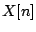, we can make other signals by time shifting the signal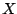by a (positive or negative) integer:so that theth sample of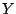is the 0th sample ofand so on. If the integeris positive, thenis a delayed copy of. Ifis negative, thenanticipates; this can be done to a recorded sound but isn't practical as a real-time operation.

Time shifting is a linear operation (considered as a function of the input signal); if you time shift a sumyou get the same result as if you time shift them separately and add afterward.

Time shifting has the further property that, if you time shift a sinusoid of frequency, the result is another sinusoid of the same frequency; time shifting never introduces frequencies that weren't present in the signal before it was shifted. This property, called time invariance, makes it easy to analyze the effects of time shifts--and linear combinations of them--by considering separately what the operations do on individual sinusoids.

Furthermore, the effect of a time shift on a sinusoid is simple: it just changes the phase. If we use a complex sinusoid, the effect is even simpler. If for instance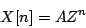thenso time shifting a complex sinusoid bysamples is the same thing as scaling it by--it's just an amplitude change by a particular complex number. Sincefor a sinusoid, the amplitude change does not change the magnitude of the sinusoid, only its phase.

The phase change is equal to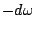, where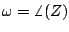is the angular frequency of the sinusoid. This is exactly what we should expect since the sinusoid advancesradians per sample and it is offset (i.e., delayed) bysamples.Next: Delay networks Up: Time shifts and delays Previous: Complex sinusoids   Contents   Index
Miller Puckette 2006-12-30